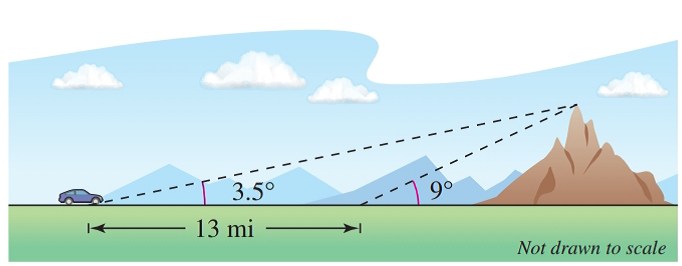Chapter 8.2, Problem 79E### Calculus: An Applied Approach (Min...

10th Edition
Ron Larson
ISBN: 9781305860919

#### Solutions

Chapter
Section### Calculus: An Applied Approach (Min...

10th Edition
Ron Larson
ISBN: 9781305860919
Textbook Problem
1 views

# Height of a Mountain In traveling across flat land, you notice a mountain directly in front of you. Its angle of elevation (to the peak) is 3.5 ° . After you drive 13 miles closer to the mountain, the angle of elevation is 9 ° (see figure). Approximate the height of the mountain.To determine

To calculate: The height of the mountain when the angle of elevation is 3.5° after driving 13 miles closer to the mountain when the angle of elevation is 9°.

Explanation

Given Information:

Initially the angle of elevation is 9° but after driving 13 miles, the angle of elevation changes to 3.5° as shown in the figure below.

Formula used:

From the definition of the right triangle,

Where opp is the length of the side opposite to angle θ and adj is the length of the side adjacent to angle θ

Calculation:

Consider the distance between point C and D is x miles as shown in below figure.

To find height AD of the mountain, use definition of tangent function in the triangle ACD.

Now, use definition of tangent function in the triangle ABD.

The distance BD is now 13+x mile after driving.

Substitute 13+x for BD.

### Still sussing out bartleby?

Check out a sample textbook solution.

See a sample solution

#### The Solution to Your Study Problems

Bartleby provides explanations to thousands of textbook problems written by our experts, many with advanced degrees!

Get Started